# IB DP Physics: HL复习笔记12.2.7 Measuring Half-Life

### Measuring Half-Life

• Half-life is defined as:

The time taken for the initial number of nuclei to halve for a particular isotope

• This means when a time equal to the half-life has passed, the activity of the sample will also half
• This is because the activity is proportional to the number of undecayed nuclei, A ∝ N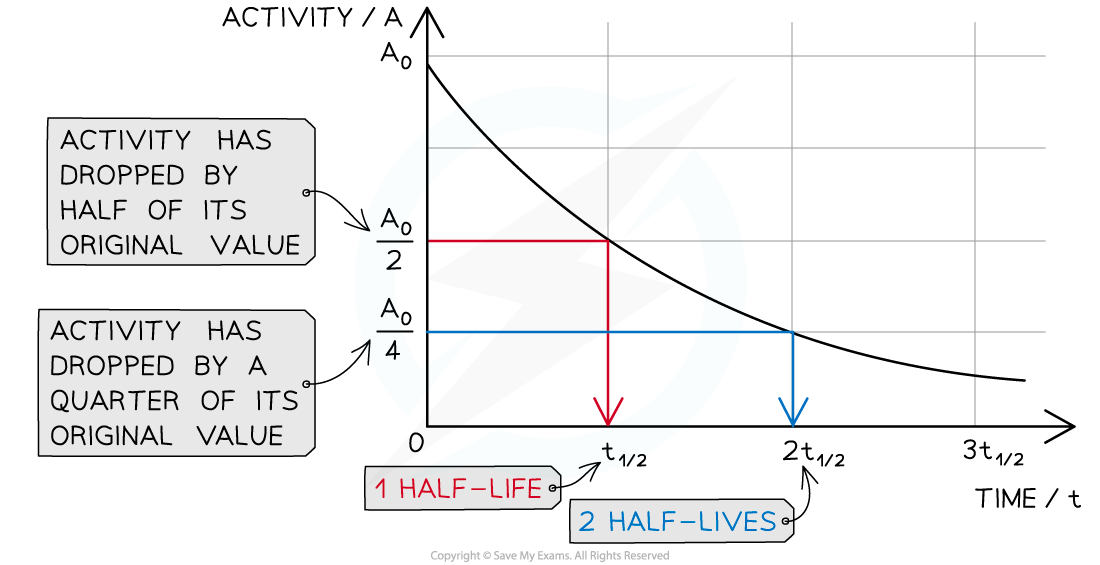When a time equal to the half-life passes, the activity falls by half, when two half-lives pass, the activity falls by another half (which is a quarter of the initial value)

• To find an expression for half-life, start with the equation for exponential decay:

N = N0 e–λt

• Where:
• N = number of nuclei remaining in a sample
• N0 = the initial number of undecayed nuclei (when t = 0)
• λ = decay constant (s-1)
• t = time interval (s)
• When time t is equal to the half-life t½, the activity N of the sample will be half of its original value, so N = ½ N0• The formula can then be derived as follows: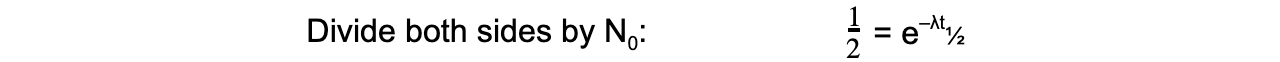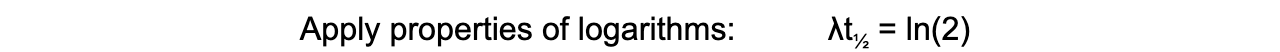• Therefore, half-life t½ can be calculated using the equation:• This equation shows that half-life t½ and the radioactive decay rate constant λ are inversely proportional
• Therefore, the shorter the half-life, the larger the decay constant and the faster the decay
• The half-life of a radioactive substance can be determined from decay curves and log graphs
• Since half-life is the time taken for the initial number of nuclei, or activity, to reduce by half, it can be found by
• Drawing a line to the curve at the point where the activity has dropped to half of its original value
• Drawing a line from the curve to the time axis, this is the half-life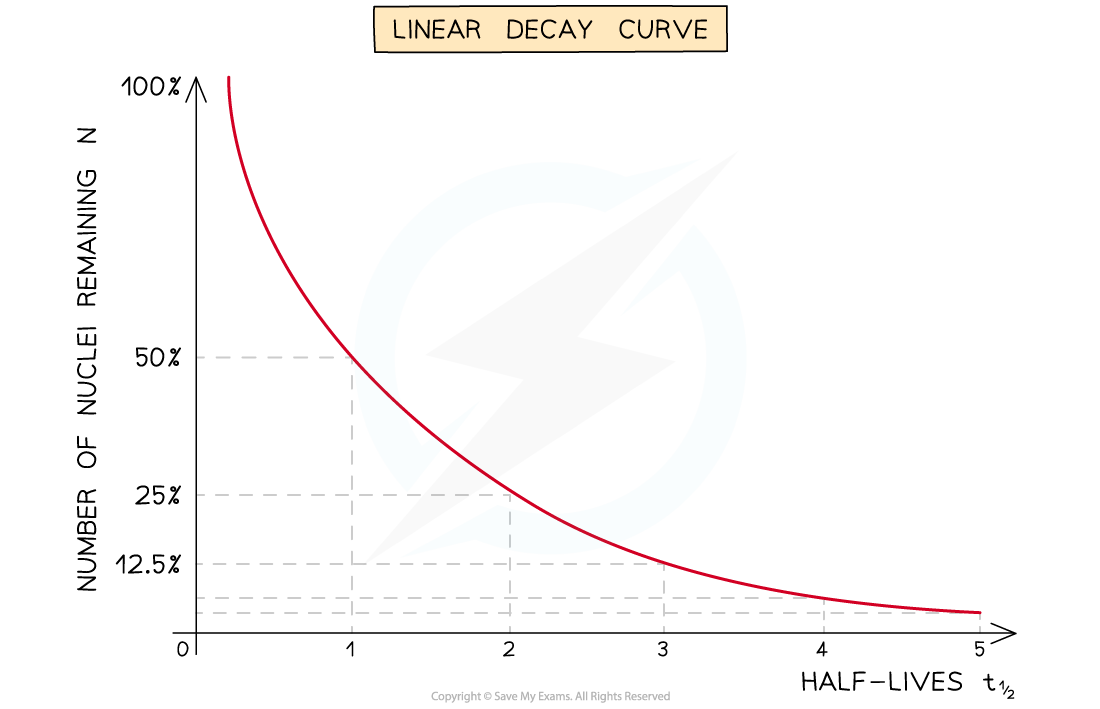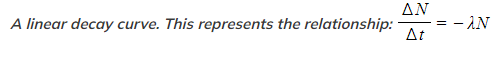Measuring Long Half-Lives

• For nuclides with long half-lives, on the scale of years, this can be measured by:
• Measuring the mass of the nuclide in a pure sample
• Determining the number of atoms N in the sample using N = nNA
• Measuring the total activity A of the sample using the counts collected by a detector
•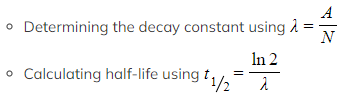Note: The sample must be sufficiently large enough in order for a significant number of decays to occur per unit time so that an accurate measure of activity can be made

#### Measuring Short Half-Lives

• For nuclides with short half-lives, on the scale of seconds, hours or days, this can be measured by:
• Measuring the background count rate in the laboratory (to subtract from each reading)
• Taking readings of the count rate against time until the value equals that of the background count rate (i.e. until all of the sample has decayed)
• Plotting a graph of activity, A, against time, t (as corrected count rate ∝ activity, A)
• Making at least 3 estimates of half-life from the graph and taking a mean

OR

• Plotting a graph of ln N against time, t (as corrected count rate ∝ number of nuclei in the sample, N)
• Finding the gradient of this graph, which gives –λ
•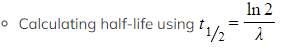Straight-line graphs tend to be more useful than curves for interpreting data
• Due to the exponential nature of radioactive decay logarithms can be used to achieve a straight line graph
• Take the exponential decay equation for the number of nuclei

N = N0 e–λt

• Taking the natural logs of both sides

ln N = ln (N0) − λt

• In this form, this equation can be compared to the equation of a straight line

y = mx + c

• Where:
• ln (N) is plotted on the y-axis
• t is plotted on the x-axis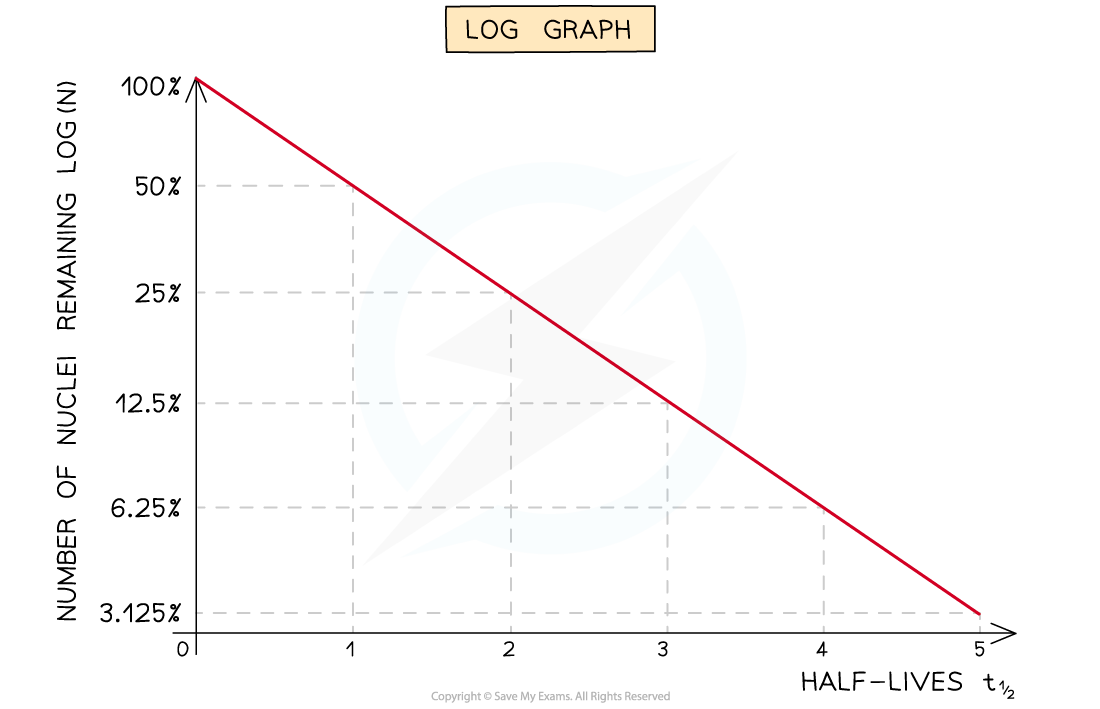A logarithmic graph. This represents the relationship: [Image_Link]data:;base64,